# Wellen Lasten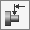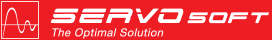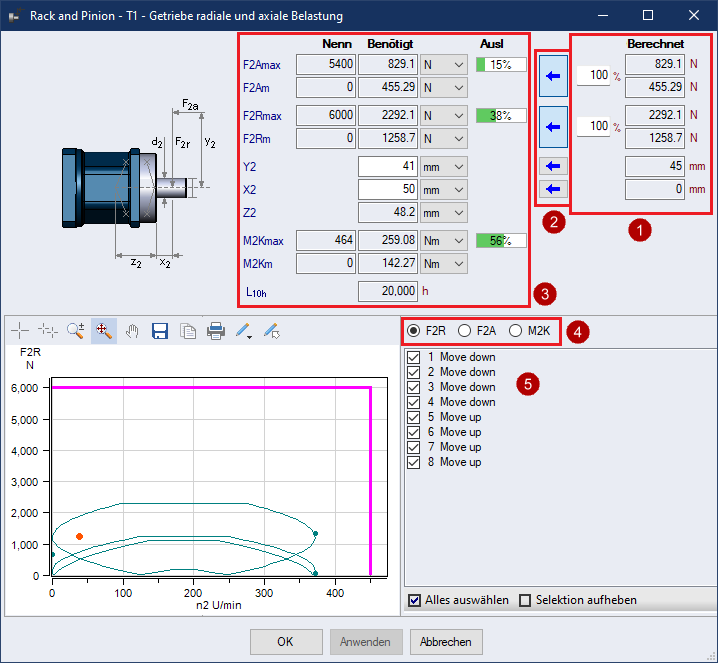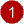Calculated Values Depending on the mechanism, radial and axial loads may be generated as well as X2 & Y2 values. For example, a Conveyor mechanism will generate a radial load [F2r], and a Rack & Pinion mechanism with a Helix Angle will generate both a radial [F2r] and axial [F2a] load. How X2 is calculated X2 = Gearbox x2 database value, which is the distance to flange-plane at output Rotary Mechanism with Shaft Loads Defined in Sequence When multiple radial forces and/or X2 values are defined in the sequence, then, an "effective" X2 value is calculated so that the proper tilting moment is applied to the output side of a gearbox connected How Y2 is calculated Rack & Pinion and Dual Rack & Conveyor: Y2 = Pinion Radius Rotary Mechanism Unbalanced Load Ratio of Unbalanced Load Mass [mUL] wrt Total Mass multiplied by the Unbalanced Load Radius [rUL] Y2 = mUL / (mUL + mDisc + mLoad) * rUL Shaft Loads Defined in Sequence When multiple axial forces and/or Y2 values are defined in the sequence, then, an "effective" Y2 value is calculated so that the proper tilting moment is applied to the output side of a gearbox connected Else Y2 = 0 How Y1 is calculated Transformation Type = Gear Y2 = Drive Gear Radius Else Y2 = 0 How X1 is calculated X1 = Gearbox x1 database value, which is the distance to flange-plane at input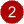Apply Calculated Values for F2a, F2r When each of the F2a and F2r buttons is selected, the corresponding calculated value(s) are applied as the application required values. When a button is not selected, the application required values can be entered manually. If the gearbox output is not exposed to any shaft loads, then de-select the F2a and F2r buttons and enter 0 for the application required values. Ie. No shaft loads are applied.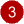Gearbox Rated and Required Values and Utilization The Rated values come from the database values, adjusted for any load factors if applicable Application Required Values can be the calculated or user specified For a Const Velocity segment, Accel and Jerk step values are also not used as Accel and Jerk are always 0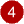Choose the profiles displayed in the chart - F2R, F2A or M2K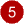Segmente im Diagramm Anzeigen / Ausblenden / Hervorheben Wenn markiert, werden die entsprechenden Profile im Diagramm angezeigt Wenn ausgewählt, werden die entsprechenden Profile im Diagramm hervorgehoben